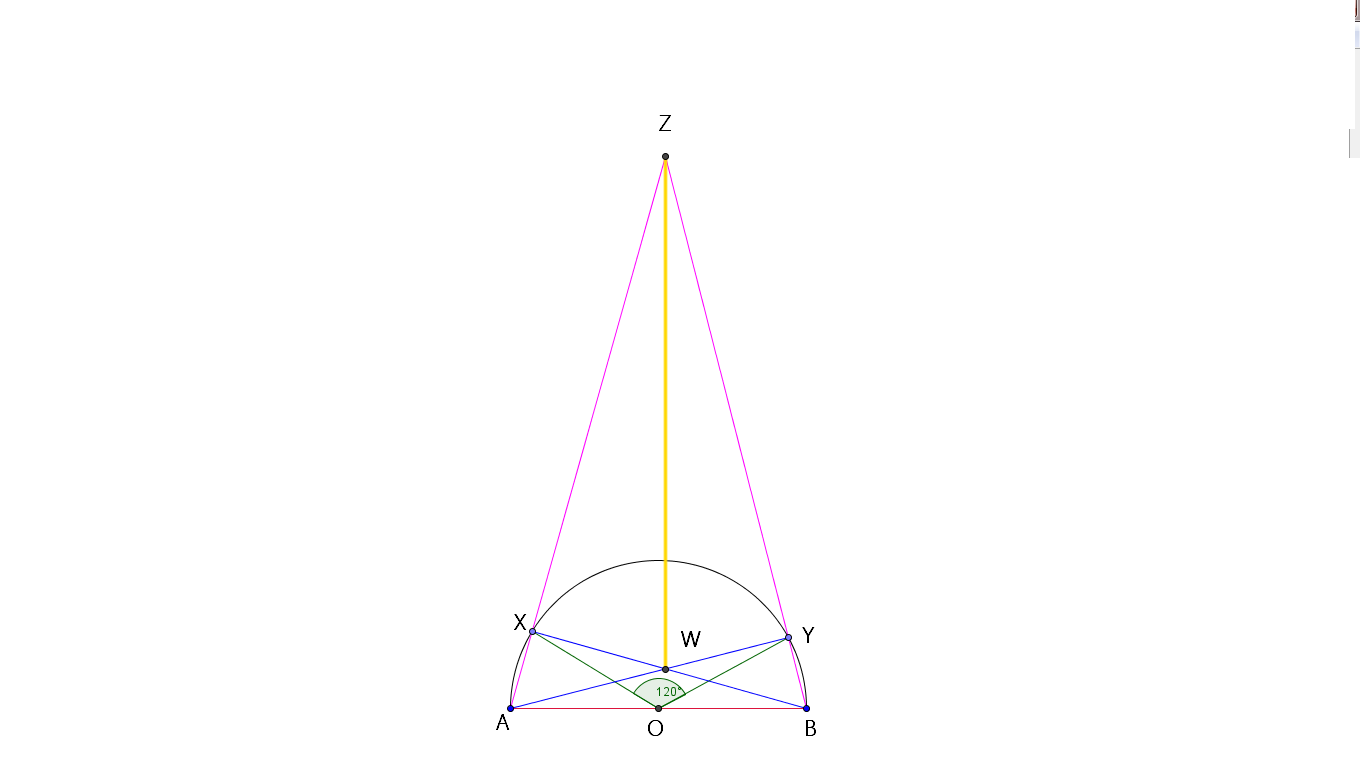# Distance Between Intersection Points

Geometry Level 4On a semicircle with diameter $AB$ and centre $O$ points $X$ and $Y$ are chosen such that $\angle XOY = 120^{\circ}$. Let $AX$ and $BY$ meet at $Z$ and $AY$ and $BX$ meet at $W$. If $AB = 5\sqrt{3}$, find $WZ$.

×

Problem Loading...

Note Loading...

Set Loading...